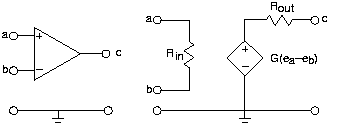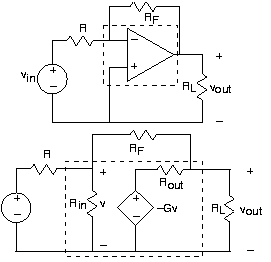# 3.19: Operational Amplifiers

$$\newcommand{\vecs}{\overset { \rightharpoonup} {\mathbf{#1}} }$$ $$\newcommand{\vecd}{\overset{-\!-\!\rightharpoonup}{\vphantom{a}\smash {#1}}}$$$$\newcommand{\id}{\mathrm{id}}$$ $$\newcommand{\Span}{\mathrm{span}}$$ $$\newcommand{\kernel}{\mathrm{null}\,}$$ $$\newcommand{\range}{\mathrm{range}\,}$$ $$\newcommand{\RealPart}{\mathrm{Re}}$$ $$\newcommand{\ImaginaryPart}{\mathrm{Im}}$$ $$\newcommand{\Argument}{\mathrm{Arg}}$$ $$\newcommand{\norm}{\| #1 \|}$$ $$\newcommand{\inner}{\langle #1, #2 \rangle}$$ $$\newcommand{\Span}{\mathrm{span}}$$ $$\newcommand{\id}{\mathrm{id}}$$ $$\newcommand{\Span}{\mathrm{span}}$$ $$\newcommand{\kernel}{\mathrm{null}\,}$$ $$\newcommand{\range}{\mathrm{range}\,}$$ $$\newcommand{\RealPart}{\mathrm{Re}}$$ $$\newcommand{\ImaginaryPart}{\mathrm{Im}}$$ $$\newcommand{\Argument}{\mathrm{Arg}}$$ $$\newcommand{\norm}{\| #1 \|}$$ $$\newcommand{\inner}{\langle #1, #2 \rangle}$$ $$\newcommand{\Span}{\mathrm{span}}$$

Learning Objectives

• A short description of the workings of an op-amp.Fig.3.19.1 The op-amp has four terminals to which connections can be made. Inputs attach to nodes a and b, and the output is node c. As the circuit model on the right shows, the op-amp serves as an amplifier for the difference of the input node voltages.

Op-amps not only have the circuit model shown in Fig. 3.19.1 above, but their element values are very special.

• The input resistance, Rin, is typically large, on the order of 1 MΩ.
• The output resistance, Rout, is small, usually less than 100 Ω.
• The voltage gain, G, is large, exceeding 105.

The large gain catches the eye; it suggests that an op-amp could turn a 1 mV input signal into a 100 V one. If you were to build such a circuit--attaching a voltage source to node a, attaching node b to the reference, and looking at the output--you would be disappointed. In dealing with electronic components, you cannot forget the unrepresented but needed power supply.

Unmodeled limitations imposed by power supplies:

It is impossible for electronic components to yield voltages that exceed those provided by the power supply or for them to yield currents that exceed the power supply's rating.

Typical power supply voltages required for op-amp circuits are + (15V). Attaching the 1 mv signal not only would fail to produce a 100 V signal, the resulting waveform would be severely distorted. While a desirable outcome if you are a rock & roll aficionado, high-quality stereos should not distort signals. Another consideration in designing circuits with op-amps is that these element values are typical: Careful control of the gain can only be obtained by choosing a circuit so that its element values dictate the resulting gain, which must be smaller than that provided by the op-amp.Fig. 3.19.2 The top circuit depicts an op-amp in a feedback amplifier configuration. On the bottom is the equivalent circuit, and integrates the op-amp circuit model into the circuit.

## Inverting Amplifier

The feedback configuration shown in Fig. 3.19.2 is the most common op-amp circuit for obtaining what is known as an inverting amplifier.

$\left ( \frac{R_{F}R_{out}}{R_{out}-GR_{F}}\left ( \frac{1}{R_{out}}+\frac{1}{R_{in}}+\frac{1}{R_{L}}\right )\left ( \frac{1}{R}+\frac{1}{R_{in}}+\frac{1}{R_{F}} \right ) -\frac{1}{R_{F}} \right )v_{out}=\frac{1}{R}v_{in}$

provides the exact input-output relationship. In choosing element values with respect to op-amp characteristics, we can simplify the expression dramatically.

• Make the load resistance, RL, much larger than Rout. This situation drops the term 1/RL from the second factor of the above equation.
• Make the resistor, R, smaller than Rin, which means that the 1/Rin term in the third factor is negligible.

With these two design criteria, the expression becomes:

$\left ( \frac{R_{F}R_{out}}{R_{out}-GR_{F}}\left ( \frac{1}{R}+\frac{1}{R_{F}} \right ) -\frac{1}{R_{F}} \right )v_{out}=\frac{1}{R}v_{in}$

Because the gain is large and the resistance Rout is small, the first term becomes -1/G, leaving us with:

$\left ( \left ( -\frac{1}{G} \right )\left ( \frac{1}{R}+\frac{1}{R_{F}} \right ) -\frac{1}{R_{F}} \right )v_{out}=\frac{1}{R}v_{in}$

If we select the values of RF and R so that GR>>RF, this factor will no longer depend on the op-amp's inherent gain, and it will equal -1/RF. Under these conditions, we obtain the classic input-output relationship for the op-amp-based inverting amplifier.

$v_{out}=-\left ( \frac{R_{F}}{R}v_{in} \right )$

Consequently, the gain provided by our circuit is entirely determined by our choice of the feedback resistor RF and the input resistor R. It is always negative, and can be less than one or greater than one in magnitude. It cannot exceed the op-amp's inherent gain and should not produce such large outputs that distortion results (remember the power supply!). Interestingly, note that this relationship does not depend on the load resistance. This effect occurs because we use load resistances large compared to the op-amp's output resistance. Thus observation means that, if careful, we can place op-amp circuits in cascade, without incurring the effect of succeeding circuits changing the behavior (transfer function) of previous ones; see this problem.

## Active Filters

As long as design requirements are met, the input-output relation for the inverting amplifier also applies when the feedback and input circuit elements are impedances (resistors, capacitors, and inductors).

$\frac{V_{out}}{V_{in}}=-\frac{Z_{F}}{Z}$

Example $$\PageIndex{1}$$:

Let's design an op-amp circuit that functions as a lowpass filter. We want the transfer function between the output and input voltage to be:

$H(f)=\frac{K}{1+\frac{if}{f_{c}}}$

where K equals the passband gain and fc is the cutoff frequency. Let's assume that the inversion (negative gain) does not matter. With the transfer function of the above op-amp circuit in mind, let's consider some choices.

• $Z_{F}=K,\; \; Z=1+\frac{if}{f_{c}}$

This choice means the feedback impedance is a resistor and that the input impedance is a series combination of an inductor and a resistor. In circuit design, we try to avoid inductors because they are physically bulkier than capacitors.

• $Z_{F}=\frac{1}{1+\frac{if}{f_{c}}},\; \; Z=\frac{1}{K}$

Consider the reciprocal of the feedback impedance (its admittance):

$Z_{F}^{-1}=1+\frac{if}{f_{c}}$

Since this admittance is a sum of admittances, this expression suggests the parallel combination of a resistor (value = 1 Ω) and a capacitor (value =(1/fc)F). We have the right idea, but the values (like 1 Ω) are not right. Consider the general RC parallel combination; its admittance is

$\frac{1}{R_{F}}+i2\pi fC$

Letting the input resistance equal R, the transfer function of the op-amp inverting amplifier now is

$H(f)=-\frac{\frac{R_{F}}{R}}{1+i2\pi fR_{F}C}$

where gain equals

$\frac{R_{F}}{R}$

and the cutoff frequency

$\frac{1}{R_{F}C}$

Creating a specific transfer function with op-amps does not have a unique answer. As opposed to design with passive circuits, electronics is more flexible (a cascade of circuits can be built so that each has little effect on the others; see [link]) and gain (increase in power and amplitude) can result. To complete our example, let's assume we want a lowpass filter that emulates what the telephone companies do. Signals transmitted over the telephone have an upper frequency limit of about 3 kHz. For the second design choice, we require RFC=5.3x10-5. Thus, many choices for resistance and capacitance values are possible. A 1 μF capacitor and a 330 Ω resistor, 10 nF and 33 kΩ, and 10 pF and 33 MΩ would all theoretically work. Let's also desire a voltage gain of ten:

$\frac{R_{F}}{R}=10$

$R=\frac{R_{F}}{10}$

Recall that we must have R<Rin.

As the op-amp's input impedance is about 1 MΩ, we don't want

$\frac{\left | Z_{F} \right |}{R}< 10^{5}$

for all frequencies of interest. Thus,

$\frac{\frac{R_{F}}{\left | 1+i2\pi fR_{F}C \right |}}{R}< 10^{5}$

As this impedance decreases with frequency, the design specification of

$\frac{R_{F}}{R}=10$

means that this criterion is easily met. Thus, the first two choices for the resistor and capacitor values (as well as many others in this range) will work well. Additional considerations like parts cost might enter into the picture. Unless you have a high-power application (this isn't one) or ask for high-precision components, costs don't depend heavily on component values as long as you stay close to standard values. For resistors, having values r10d, easily obtained values of

Exercise $$\PageIndex{1}$$

What is special about the resistor values; why these rather odd-appearing values for r?

Solution

The ratio between adjacent values is about $\sqrt{2}$

## Intuitive Way of Solving Op-Amp Circuits

When we meet op-amp design specifications, we can simplify our circuit calculations greatly, so much so that we don't need the op-amp's circuit model to determine the transfer function. Here is our inverting amplifier.

When we take advantage of the op-amp's characteristics—large input impedance, large gain, and small output impedance—we note the two following important facts.

• The current iin must be very small. The voltage produced by the dependent source is 105 times the voltage v. Thus, the voltage
• Because of this assumption—essentially no current flow through Rin —the voltage

Armed with these approximations, let's return to our original circuit as shown in Fig. 3.19.4. The node voltage e is essentially zero, meaning that it is essentially tied to the reference node. Thus, the current through the resistor R is:

$i=\frac{v_{in}}{R}$

Furthermore, the feedback resistor appears in parallel with the load resistor. Because the current going into the op-amp is zero, all of the current flowing through R flows through the feedback resistor (iF=i)! The voltage across the feedback resistor R equals:

$v=\frac{v_{in}R_{F}}{R}$

Because the left end of the feedback resistor is essentially attached to the reference node, the voltage across it equals the negative of that across the output resistor:

$v_{out}=-v=-\frac{v_{in}R_{F}}{R}$

Using this approach makes analyzing new op-amp circuits much easier. When using this technique, check to make sure the results you obtain are consistent with the assumptions of essentially zero current entering the op-amp and nearly zero voltage across the op-amp's inputs.

Example $$\PageIndex{1}$$:

Let's try this analysis technique on a simple extension of the inverting amplifier configuration shown in the above Fig. 3.19.5. If either of the source-resistor combinations were not present, the inverting amplifier remains, and we know that transfer function. By superposition, we know that the input-output relation is:

$v_{out}=\left ( -\left ( \frac{R_{F}}{R_{1}} v_{in}^{(1)}\right ) \right )-\frac{R_{F}}{R_{2}} v_{in}^{(2)}$

When we start from scratch, the node joining the three resistors is at the same potential as the reference,

$e\simeq 0$

The sum of currents flowing into that node is zero. Thus, the current i flowing in the resistor RF equals:

$i=\frac{v_{in}^{(1)}}{R_{1}}+\frac{v_{in}^{(2)}}{R_{2}}$

Because the feedback resistor is essentially in parallel with the load resistor, the voltages must satisfy

$v=-v_{out}$

In this way, we obtain the input-output relation given above.

What utility does this circuit have? Can the basic notion of the circuit be extended without bound?

## Contributor

• ContribEEOpenStax

3.19: Operational Amplifiers is shared under a CC BY license and was authored, remixed, and/or curated by LibreTexts.